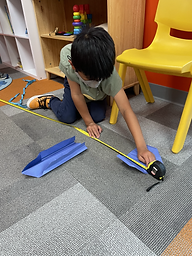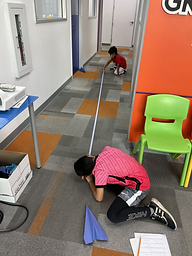## Ms. Caitlyn

### Target 1​

###### Lesson Type:

Continuation

Number Operation

:

Computation

Divide within 1,000.

###### 1:

Understand that there are multiple ways to divide multi-digit numbers.

###### 2:

Understand the role of place-value in division strategies.

###### 3:

Use the standard algorithm for solving multi-digit division equations.

###### 4:

Understand that there can be a remainder in a division problem.

5th

###### Vocabulary:

Divisor, Dividend, Quotient, Remainder

Activities:

Students played a board game called "Bump," where they rolled dice to solve division problems and cover spaces on the board.### Home Exploration

###### Guiding Questions:## Absent Students:

Alejandro

### Target 2

:

###### 1:

Understand equivalent measurements.

###### 2:

Understand how to convert from one unit to another.

5th

###### Vocabulary:

Unit Conversion, Unit of Measurement, Metric System

Activities:

Students flew paper airplanes and measured their distances in inches and centimeters, then converted them to meters, yards, and feet.### Home Exploration

Students were sent home with the remaining problems on their conversion sheets to practice.

Allow students to have a conversion key.

Ask questions like, "How many meters are in a centimeter?" to prompt them to think about how to convert.

###### Guiding Questions:### Target 3

:

###### Vocabulary:

Activities:### Home Exploration